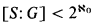Hostname: page-component-797576ffbb-58z7q Total loading time: 0 Render date: 2023-12-09T18:13:47.159Z Has data issue: false Feature Flags: { "corePageComponentGetUserInfoFromSharedSession": true, "coreDisableEcommerce": false, "useRatesEcommerce": true } hasContentIssue false

# Subgroups of small index in infinite symmetric groups. II

Published online by Cambridge University Press:  12 March 2014

## Extract

Throughout this paper κ denotes an infinite cardinal, S = Sym(κ) and G is a subgroup of S. We shall be seeking the subgroups G with [S: G] < 2κ. In , the following result was proved.

Theorem 1. If [S: G] ≤ κthen there exists a subset Δ of k such that ∣Δ∣ < k and S(Δ) ≤ G.

Here S(Δ) = Sym(K/⊿) is the pointwise stabilizer of Δ in S.

However, the converse of Theorem 1 is not true. For if cf(κ) ≤ ∣Δ∣ < κ, then [S: S(Δ)] ≥ κcf(κ) > κ. This suggests that a substantially sharpened version of Theorem 1 may be true.

Question 1 . Is it provable in ZFC, or even in ZFC with GCH, that if [S: G] ≤ κ then there is a subset Δ of κ such that ∣Δ∣ < cf(κ) and S(Δ)G?

At least two of the authors of  made a serious attempt to answer the above question positively. In §3, we shall see that they were essentially trying to prove that measurable cardinals do not exist.

The following result, due independently to Semmes  and Neumann , suggests a second way in which Theorem 1 might be improved.

Theorem 2. If k = ℵ0andthen there is a finite subset Δ of k such thatS(Δ) ≤ G.

Question 2 . Is it provable in ZFC that if [S: G] < 2κ then there is a subset Δ of κ such that ∣Δ∣ < κ and S(Δ) < G?

This question will be answered negatively in §4.

Type
Research Article
Information
The Journal of Symbolic Logic , March 1989 , pp. 95 - 99

## Access options

Get access to the full version of this content by using one of the access options below. (Log in options will check for institutional or personal access. Content may require purchase if you do not have access.)

## References

#### REFERENCES

Ben-David, S. and Magidor, M., The weak □* is really weaker than the full □, this Journal, vol. 51 (1986), pp. 10291033.Google Scholar
Dixon, J. D., Neumann, P.M. and Thomas, S., Subgroups of small index in infinite symmetric groups, Bulletin of the London Mathematical Society, vol. 18 (1986), pp. 580586.Google Scholar
Donder, H. D., Regularity and decomposability of uniform ultrafilters (to appear).Google Scholar
Kunen, K., Set theory, North-Holland, Amsterdam, 1980.Google Scholar
Semmes, S. W., Endomorphisms of infinite symmetric groups, Abstracts of Papers Presented to the American Mathematical Society, vol. 2 (1981), p. 426.Google Scholar
Semmes, S. W., Infinite symmetric groups, maximal subgroups and filters, Abstracts of Papers Presented to the American Mathematical Society, vol. 3 (1982), p. 38.Google Scholar
Williams, N. H., Combinatorial set theory, North-Holland, Amsterdam, 1977.Google Scholar